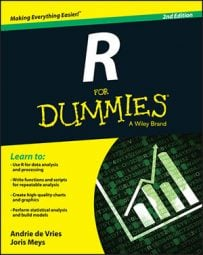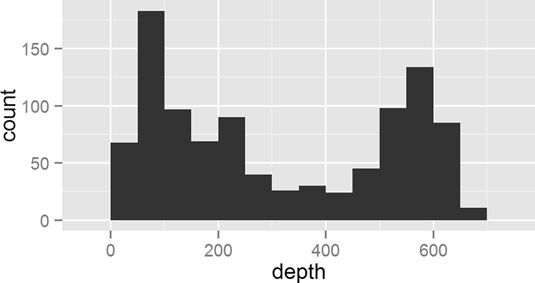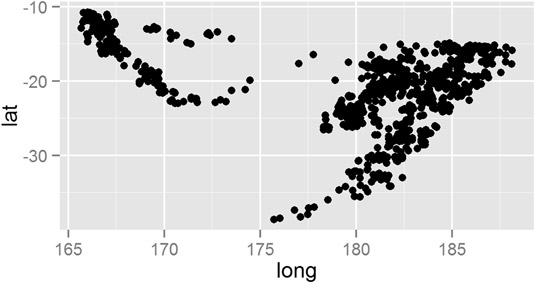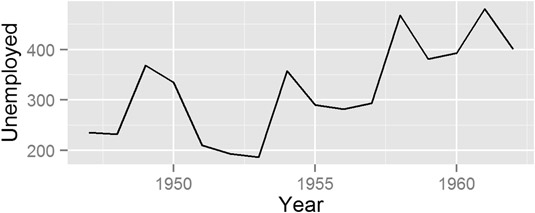##### R For DummiesA ggplot2 geom tells the plot how you want to display your data in R. For example, you use geom_bar() to make a bar chart. In ggplot2, you can use a variety of predefined geoms to make standard types of plot.

A geom defines the layout of a ggplot2 layer. For example, you can use geoms to create bar charts, scatterplots, and line diagrams (as well as a variety of other plots), as you can see below.

Each geom has a default stat, and each stat has a default geom. In practice, you have to specify only one of these.

Geom Description Default Stat
geom_bar() Bar chart stat_bin()
geom_point() Scatterplot stat_identity()
geom_line() Line diagram, connecting observations in order by x-value stat_identity()
geom_boxplot Box-and-whisker plot stat_boxplot()
geom_path Line diagram, connecting observations in original order stat_identity()
geom_smooth Add a smoothed conditioned mean stat_smooth()
geom_histogram An alias for geom_bar() and stat_bin() stat_bin()

## Creating a bar chart

To make a bar chart, you use the geom_bar() function. However, note that the default stat is stat_bin(), which is used to cut your data into bins. Thus, the default behavior of geom_bar() is to create a histogram.

For example, to create a histogram of the depth of earthquakes in the quakes dataset, try:

```> ggplot(quakes, aes(x = depth)) + geom_bar()
> ggplot(quakes, aes(x = depth)) + geom_bar(binwidth = 50)```

Notice that your mapping defines only the x-axis variable (in this case, quakes\$depth). A useful argument to geom_bar() is binwidth, which controls the size of the bins that your data is cut into. This creates this plot.geom_bar()." width="535"/>
Making a histogram with geom_bar().

So, if geom_bar() makes a histogram by default, how do you make a bar chart? The answer is that you first have to aggregate your data, and then specify the argument stat=identity in your call to geom_bar().

In the next example, you use aggregate() to calculate the number of quakes at different depth strata:

```> quakes.agg <- aggregate(mag ~ round(depth, -1), data = quakes,
+             FUN = length)
> names(quakes.agg) <- c("depth", "mag")```

Now you can plot the object quakes.agg with geom_bar(stat=identity):

```> ggplot(quakes.agg, aes(x = depth, y = mag)) +
+  geom_bar(stat = "identity")```

In summary, you can use geom_bar() to create a histogram and let ggplot2 summarize your data, or you can summarize your data and then use stat=identity to plot a bar chart.

## Making a scatterplot

To create a scatterplot, you use the geom_point() function. A scatterplot creates points (or sometimes bubbles or other symbols) on your chart. Each point corresponds to an observation in your data.

You’ve probably seen or created this type of graphic a million times, so you already know that scatterplots use the Cartesian coordinate system, where one variable is mapped to the x-axis and a second variable is mapped to the y-axis.

In exactly the same way, in ggplot2 you create a mapping between x-axis and y-axis variables. So, to create a plot of the quakes data, you map quakes\$long to the x-axis and quakes\$lat to the y-axis:

`> ggplot(quakes, aes(x = long, y = lat)) + geom_point()`

This creates this scatterplot.geom_point()." width="535"/>
Making a scatterplot with geom_point().

## Creating line charts

To create a line chart, you use the geom_line() function. You use this function in a very similar way to geom_point(); the difference is that geom_line() draws a line between consecutive points in your data.

This type of chart is useful for time series data in data frames, such as the population data in the built-in dataset longley. To create a line chart of unemployment figures, you use the following:

`> ggplot(longley, aes(x = Year, y = Unemployed)) + geom_line()`

This creates this line.geom_line()." width="535"/>
Drawing a line chart with geom_line().

You can use either geom_line() or geom_path() to create a line drawing in ggplot2. The difference is that geom_line() first orders the observations according to x-value, whereas geom_path() draws the observations in the order found in the data.